10.3 Taylor Series Error#### Find the 6th degree approximation for cos(1) centered at x = 0 and compute the error of the estimate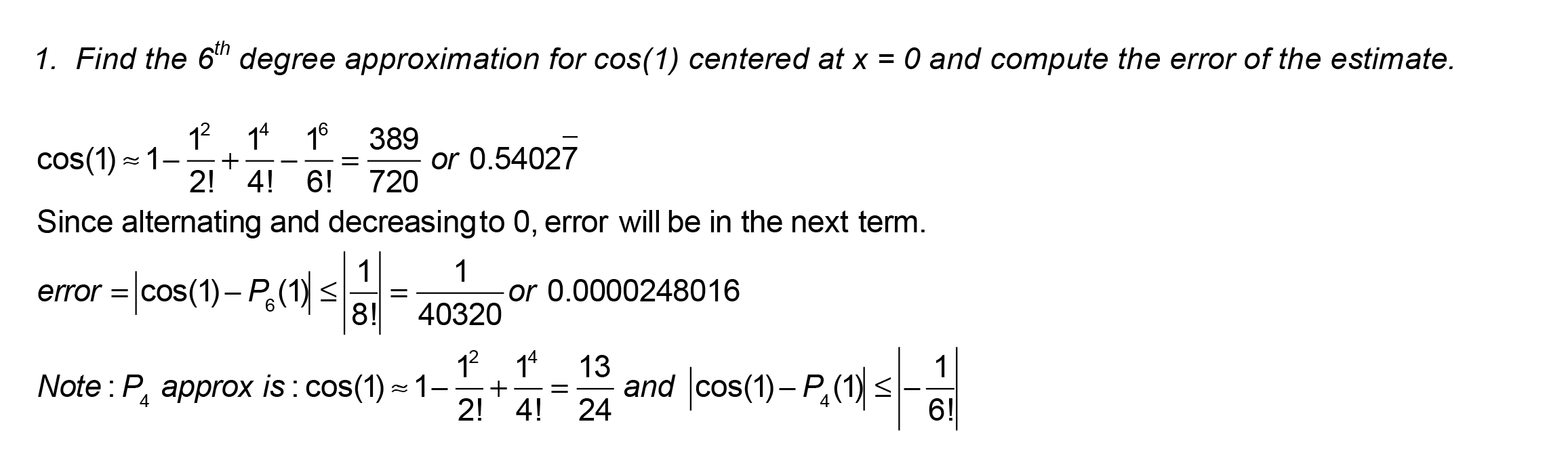#### Find the 5th degree approximation for ln(1.5) and compute the error of the estimate.#### Given g^(n) (3)=(-2/3)^n find the T3(x) Taylor approximation centered at x = 3.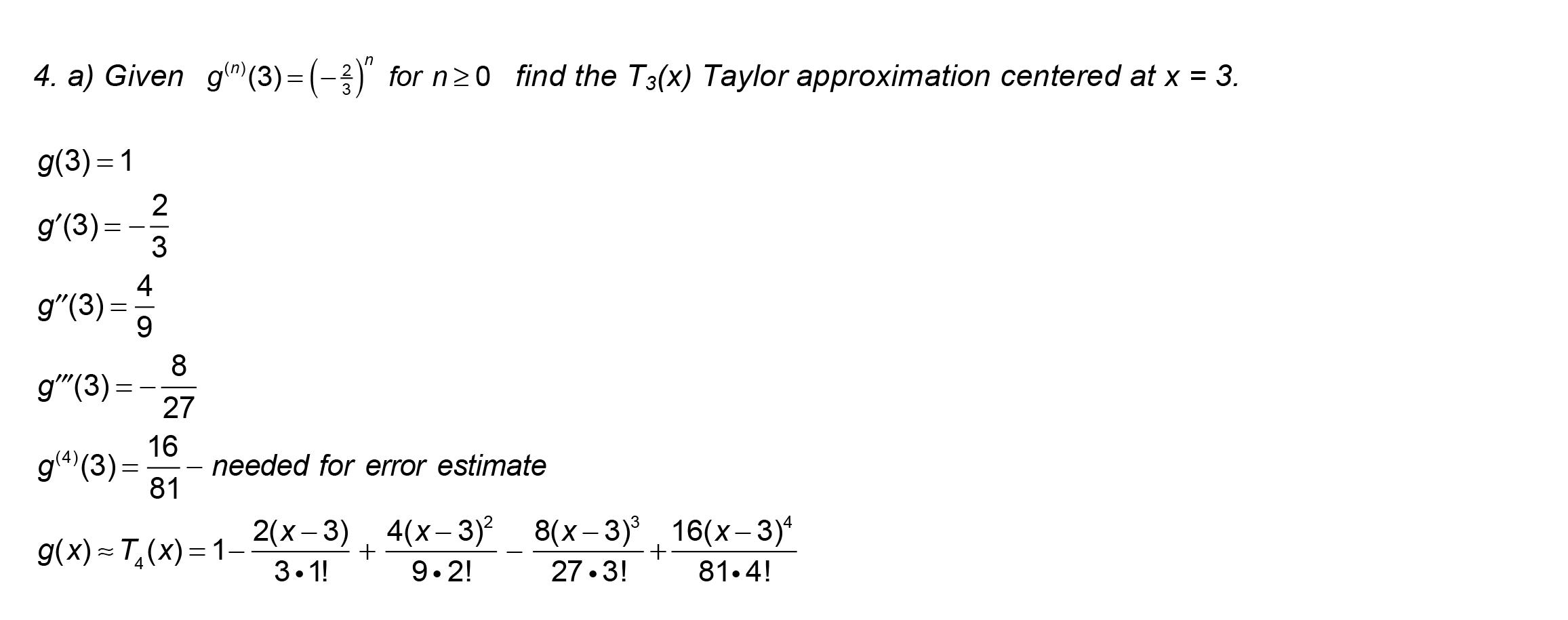#### Use the T3(x) found above to approximate g(3.2) and compute the error of the estimate, if possible.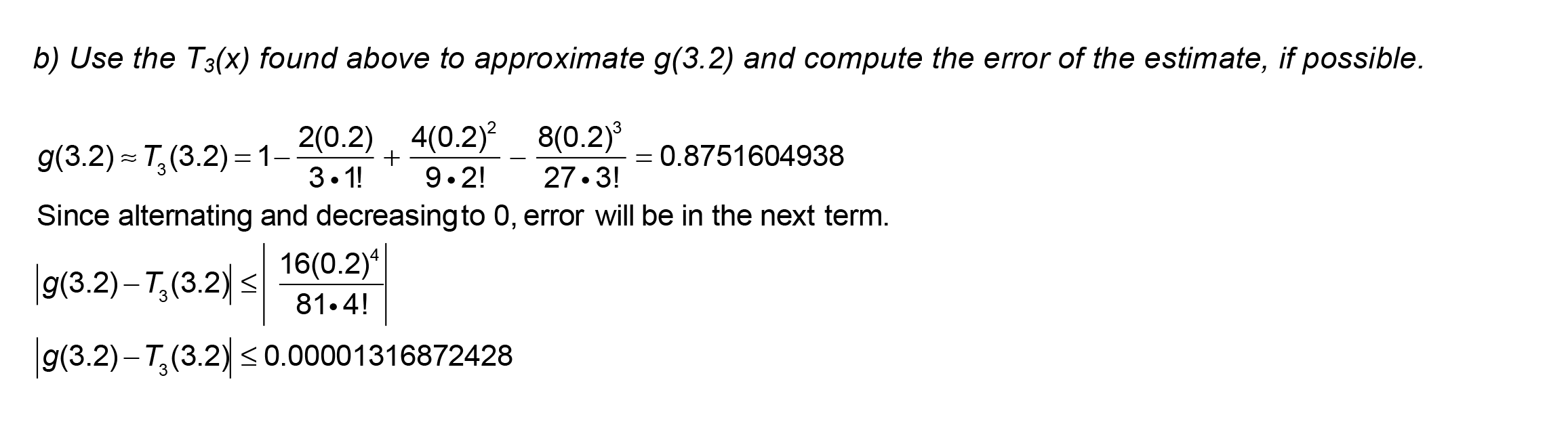#### Find the approximation of the T4(x) Taylor polynomial centered at x = 0 for 1/e and then find the error.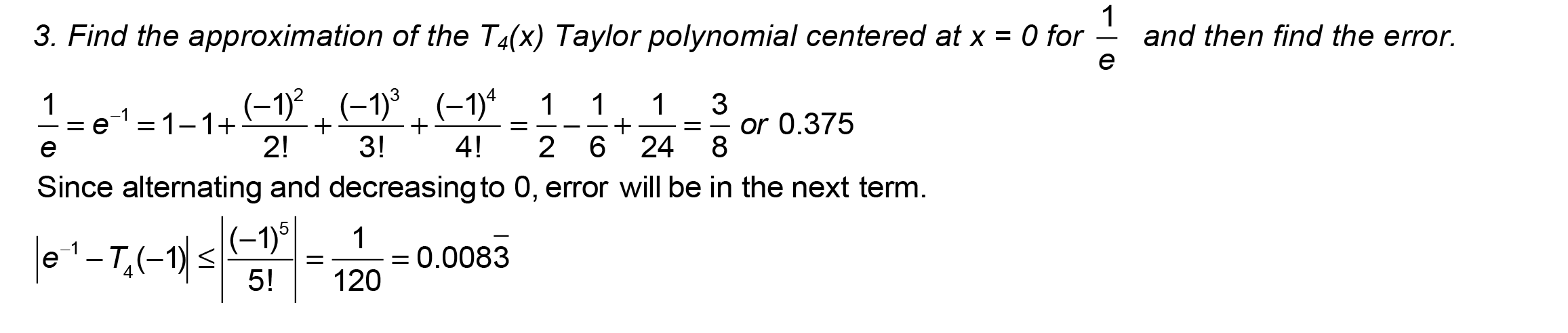#### Using the approximation of the T4(x) Taylor polynomial centered at x = 0 for 1/e, how many terms would be necessary so that the error is within 0.0001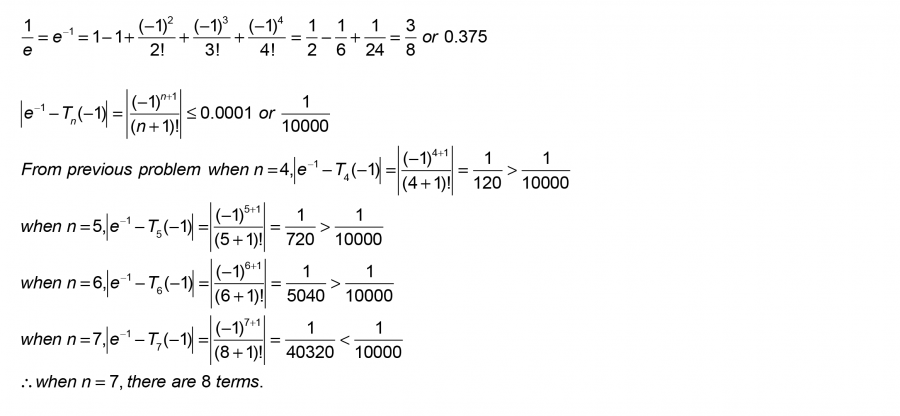#### Find the P4 Taylor series for f(x) centered at x = 0 if f(x) = sqrt(x + 1)#### Find approximation at x = -½ and compute the error.#### Find the P4 Taylor series for g(x) centered at x = 1 if g(x) = ln(x)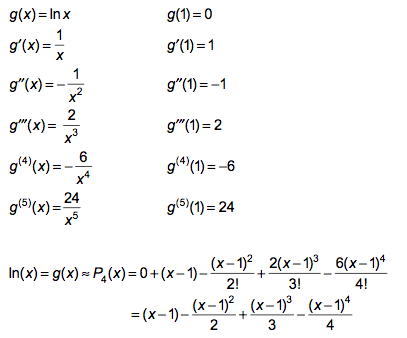#### Find approximation at x = ½ and compute the error.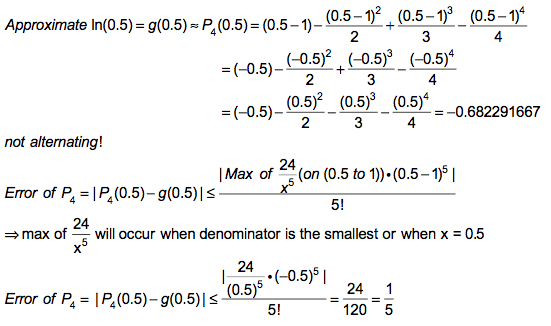#### Use the first 5 terms of the Maclaurin series for e^x. Use it to find an approximation for e and the error!#### Given